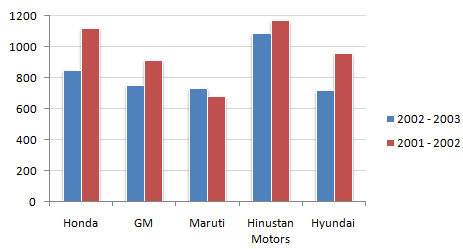# Data Interpretation - Bar Charts - Discussion

Study the following bar chart and answer the questions carefully.

Sales Turnover of 5 Companies (in Rs. crores)2.

What is the absolute change in overall sales turnover of the five companies together between 2001 - 2002 and 2002 - 2003 ?

 [A]. 712.43 [B]. 142.48 [C]. 683.53 [D]. None of these

Explanation:

Absolute value of the difference between the sum of the turnover of the five companies for 2001 - 2002 and 2002 - 2003.

 Krishnam Naidu said: (Mar 15, 2013) What is the difference between percentage change and absolute change? explain?

 Spr said: (Dec 29, 2013) @Faud - You need to find the sales turnover as accurate to scale as possible. Hence the crazy answer with two digits after decimal point. @Naidu - (X-Y)is the absolute change between X and Y. ((X-Y)/X)*100 is the percentage change (or relative change).

 Pradeep said: (Apr 26, 2014) From question (i), it's absolutely 4750-4050 = 700. Not sure, how the crazy answer 712.43% come.

 Shubham said: (Mar 18, 2015) If 800 is absolute change, then how could 712.43 be the answer. We can choose option D.

 Raja said: (Apr 18, 2017) How the answer is option A? explain it.

 Nishtha said: (Dec 16, 2017) As the difference is 700, hence taking the near most value 712.43.

 Suchilita said: (Jul 26, 2018) Absolute change = (sum of all the values of 2001-02) - (sum of all the values of 2002-03).

 Dilip Kumar said: (Sep 28, 2019) The answer should be 700 not 712.43. but as we can see the data is not accurate as we are taking like we are taking 800 first value but that is not 800.

 Deepu said: (Mar 15, 2020) Option D is the right one. We can't take A because too much gap is there 12.43.

 Joseph said: (Jul 17, 2020) Yes, Option D is valid. We just estimated some values because the graph is not to giving distinct values.